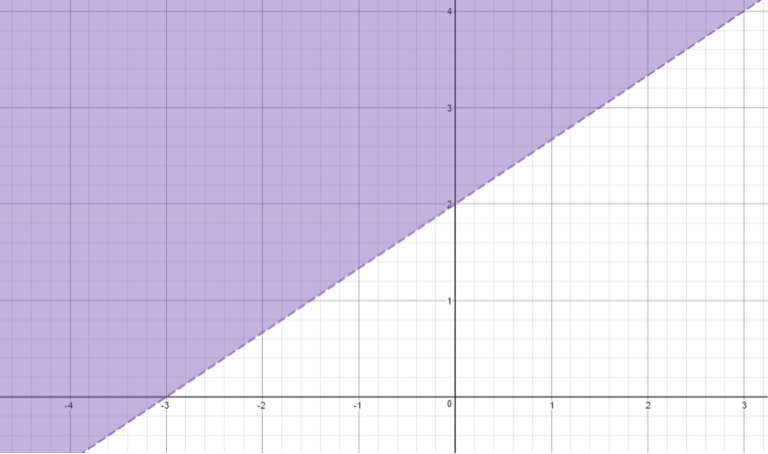# LINR 2 | Lesson 3 | Try This!

Let’s look at how we can determine the shading of an inequality and the meaning of the dashing of the border line.

For the following graph:  The equation of the line is $$2x-3y =-6$$

A linear inequality divides the plane into 3 parts: the line, and the regions on either side of the line. In the graph above, the line can be represented by

One student decided that because the shading is above the line. How can we tell if she was correct? Graph created using Desmos.com.

• Find an appropriate rule.
• How you can verify the accuracy of your rule.##### Check solution here.